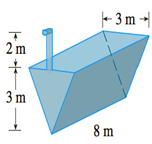Chapter 5.4, Problem 23E

Chapter
Section
Textbook Problem

# A tank is full of water. Find the work required to pump the water out of the spout. In Exercises 25 and 26 use the fact that water weighs 62.5  lb/ft 3 .To determine

To find:

The work required to pump the water out of the spout.

Explanation

1) Concept:

The work is calculated by using the formula W=abf(x)dx

And weight=gravity·volume

2) Given:

3) Calculation:

From the given figure, observe that the top of the given tank is rectangular in shape.

Let a rectangular slice of water at height x m from bottom have thickness =x m

We need to find the width of the slice at x m from bottom. Let it be w. Then by similar triangles w/x= 3/3.So the width of slice at x m height is =x m

Volume of the slice =8x x m3

and weight of the slice is =(9.8×1000)(8xx)N.Because 1 meter cube of water has 1000 kg mass.

This slice of water must be lifted (5-x) m by the pump.

Therefore, work needed to life this slice is 9.8×10008xx(5-x) m  J.

andtherefore, the total work required is

W=abf(x)dx

W039

### Still sussing out bartleby?

Check out a sample textbook solution.

See a sample solution

#### The Solution to Your Study Problems

Bartleby provides explanations to thousands of textbook problems written by our experts, many with advanced degrees!

Get Started

#### In Exercise 11-14, factor the expression. x2+5x+6

Calculus: An Applied Approach (MindTap Course List)

#### In Exercises 69-74, rationalize the numerator. 73. x2z3y

Applied Calculus for the Managerial, Life, and Social Sciences: A Brief Approach

#### In Exercises 110, find the graphical solution of each inequality. 4x3y24

Finite Mathematics for the Managerial, Life, and Social Sciences